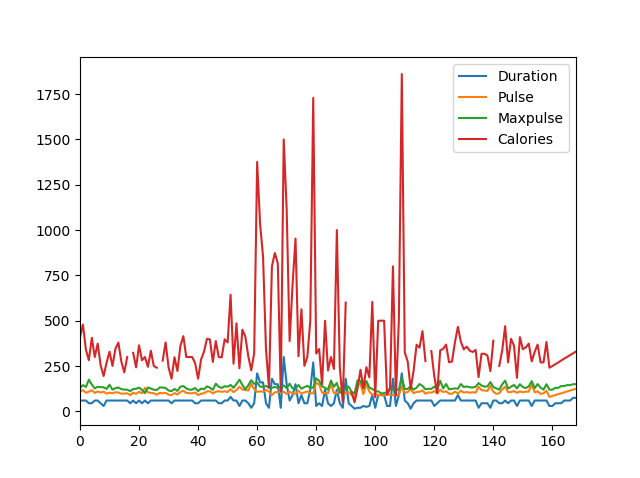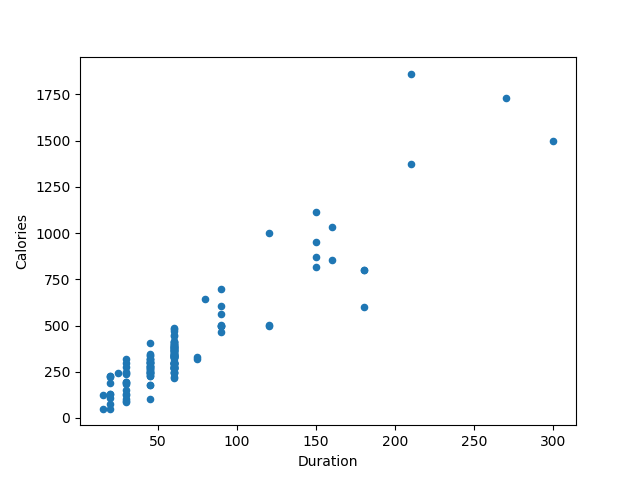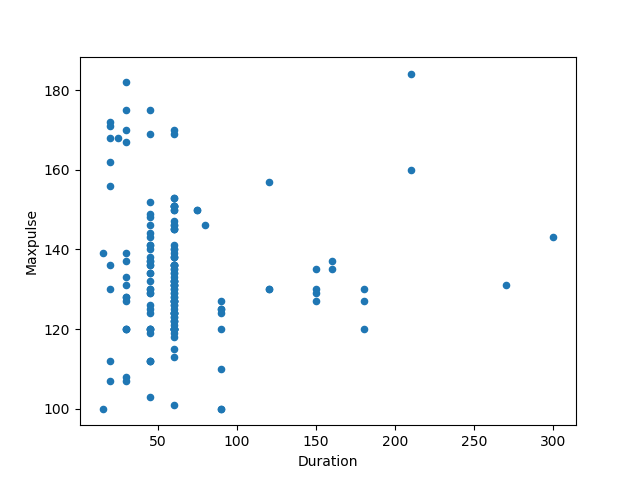# Pandas – Plotting## Plotting

Pandas uses the `plot()` method to create diagrams.

We can use Pyplot, a submodule of the Matplotlib library to visualize the diagram on the screen.

### Example

Import pyplot from Matplotlib and visualize our DataFrame:

Try it Yourself »

## Scatter Plot

Specify that you want a scatter plot with the `kind` argument:

`kind = 'scatter'`

A scatter plot needs an x- and a y-axis.

In the example below we will use “Duration” for the x-axis and “Calories” for the y-axis.

Include the x and y arguments like this:

`x = 'Duration', y = 'Calories'`

### ResultTry it Yourself »

Remember: In the previous example, we learned that the correlation between “Duration” and “Calories” was `0.922721`, and we conluded with the fact that higher duration means more calories burned.

By looking at the scatterplot, I will agree.

Let’s create another scatterplot, where there is a bad relationship between the columns, like “Duration” and “Maxpulse”, with the correlation `0.009403`:

### Example

A scatterplot where there are no relationship between the columns:

### ResultTry it Yourself »